# Pandas Coalesce - How to Replace NaN values in a dataframe

In this post we will discuss on how to use fillna function and how to use SQL coalesce function with Pandas, For those who doesn’t know about coalesce function, it is used to replace the null values in a column with other column values. Also in some cases you want to create a new column with values filled-in from another column and if any of the values are null in that column then it should be replaced by the next column value. I think after going through the below examples it will be more clear on how and when to use the Coalesce Function.

## What is Coalesce?

Suppose you have a table with three different rates for the workers i.e. Hourly,Daily and Weekly Rate and you want to calculate the wages of these workers at the end of a month and for that you want to know the rate for each of these workers, if Hourly rate is missing then apply Daily rate and if Daily is missing then apply Weekly.

### Original Table: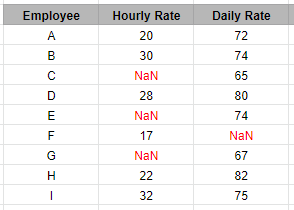### Expected Result:

A column Final Rate is inserted which contains the Hourly rate and if any values is NaN then it is replaced by the Daily Rate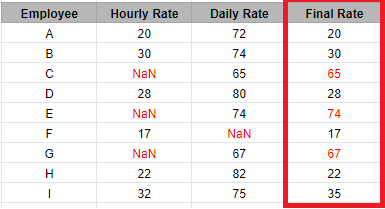For employee C Hourly Rate is null and that’s why we filled that with his Daily Rate i.e. 65 and Similarly For Employee E Hourly rate is missing so Final rate is Daily rate i.e. 74 and Same for employee G

## Using Coalesce in Pandas

Lets take a look at the different ways how you can use coalesce in Pandas using the same above example of Hourly and Daily Rate

### Create Dataframe

``````import pandas as pd
import numpy as np

df = pd.DataFrame({
'Hourly Rate': [20, 30, np.nan, 28, np.nan, 17, np.nan,22, 32],
'Daily Rate': [72, 74,65,80, 74, np.nan, 67,82,75]})
df
``````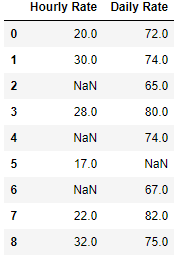The mask method is an application of the if-then idiom. For each element in the calling DataFrame, if condition is False the element is used; otherwise the corresponding element from the DataFrame other is used.

``````df['Final Rate']=df['Hourly Rate'].mask(pd.isnull, df['Daily Rate'])
df
``````

### Using Numpy

This works exactly the same way as if-else, if condition is True then first parameter is returned else the second one, So in this case if Hourly Rate is null then Daily Rate is returned else Hourly Rate

``````df['Final Rate'] = np.where(df['Hourly Rate'].isnull(),df['Daily Rate'],df['Hourly Rate'])
``````

### Using Assign

It is basically used to `assign` a new column to an existing dataframe and `lookup` is used to return a label based indexing dataframe. Using those index find if any of the value is null then replace that with the first minimum value encountered in that row using idxmin.

``````df.assign(Final_Rate=df.lookup(df.index, df.isnull().idxmin(1)))
``````

### Final Result: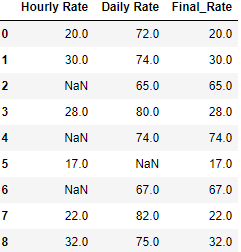So far we have seen what are the different ways Coalesce can be used in Pandas. In the next section we will see how to fill the NaN values in a column by creating a new dataframe object using fillna - bfill and ffill. This would be quite helpful when you don’t want to create a new column and want to update the NaN within the same dataframe with previous and next row and column values

## How pandas bfill works?

bfill is a method that is used with fillna function to back fill the values in a dataframe. so if there is a NaN cell then bfill will replace that NaN value with the next row or column based on the axis 0 or 1 that you choose. Let’s see how it works. First create a dataframe with those 3 columns Hourly Rate, Daily Rate and Weekly Rate

``````import pandas as pd
import numpy as np

df = pd.DataFrame({
'Hourly Rate': [20, 30, np.nan, 28, np.nan, 17, np.nan,22, 32],
'Daily Rate': [72, 74,65,80, np.nan, 74,67,82,75],
'Weekly Rate': [250,290,340,325,345,315,np.nan,248,265]})
df
``````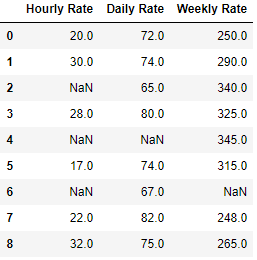Next we will fill all those NaN values with the value from next row data

``````df.bfill(axis=0)
``````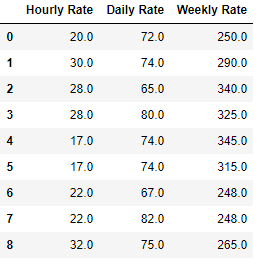Use axis=1 if you want to fill the NaN values with next column data

## How pandas ffill works?

ffill is a method that is used with fillna function to forward fill the values in a dataframe. so if there is a NaN cell then ffill will replace that NaN value with the next row or column based on the axis 0 or 1 that you choose. Let’s see how it works.

We will use the same dataframe as in bfill section above and we will now fill that dataframe NaN values with the previous row data

``````df.ffill(axis=0)
``````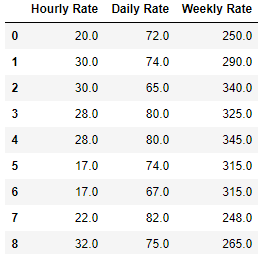Use axis=1 if you want to fill the NaN values with next column data

## Fillna with specific column

Let’s look at a unique problem which is same as the problem we solved above but we have three columns this time i.e Hourly, Daily and Weekly rates and we want to create a new column called as Final Rate, which will primarily have an Hourly rate but if Hourly is missing then will be filled by Daily or Weekly Rate of the same row

### Create Dataframe

``````import pandas as pd
import numpy as np

df = pd.DataFrame({
'Hourly Rate': [20, 30, np.nan, 28, np.nan, 17, np.nan,22, 32],
'Daily Rate': [72, 74,65,80, np.nan, 74,67,82,75],
'Weekly Rate': [250,290,340,325,345,315,np.nan,248,265]})
df
``````### Use Fillna

We have first created the final rate column with all values NaN in it and then using fillna function we have replaced Hourly rate with Daily and Daily with weekly if NaN

``````df['Final Rate'] = np.NaN
df['Final Rate'] = df['Hourly Rate'].fillna(df['Hourly Rate']).fillna(df['Daily Rate']).fillna(df['Weekly Rate'])
df
``````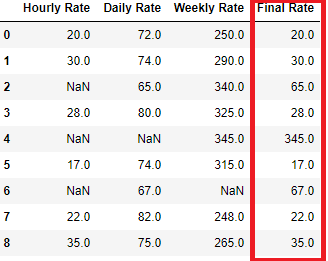## Replace NaN with the mean using fillna

Sometime you want to replace the NaN values with the mean or median or any other stats value of that column instead replacing them with prev/next row or column data. fillna function gives the flexibility to do that as well. Let’s see how we can do that

``````df.fillna(df.mean())
``````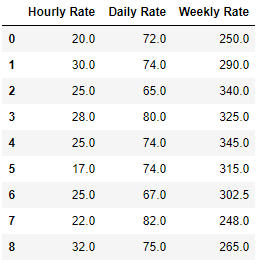## Conclusion

In this post we have seen what are the different ways we can apply the coalesce function in Pandas and how we can replace the NaN values in a dataframe. Pandas gives enough flexibility to handle the Null values in the data and you can fill or replace that with next or previous row and column data. You can also fill the value with the column mean, median or any other stats value.

Tags:

Categories:

Updated: